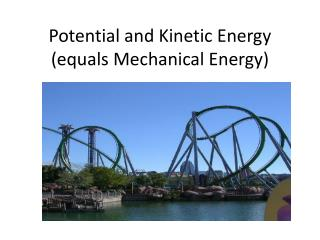DownloadDownload PresentationPotential and Kinetic Energy (equals Mechanical Energy)

# Potential and Kinetic Energy (equals Mechanical Energy)

Download Presentation## Potential and Kinetic Energy (equals Mechanical Energy)

- - - - - - - - - - - - - - - - - - - - - - - - - - - E N D - - - - - - - - - - - - - - - - - - - - - - - - - - -
##### Presentation Transcript

1. Potential and Kinetic Energy (equals Mechanical Energy)

2. Energy -A measure of the ability to change or move matter -Is measured in Joules (J)- hmm….just like work! -Whenever work is done energy is used

3. Potential Energy • Energy stored in an object is called Potential Energy • There are Two Types of Potential Energy – • Elastic Potential Energy: Potential Energy Stored in a stretched object (i.e. rubber band, bungy cord, etc)

4. Potential Energy • Gravitational Potential Energy: Potential energy an object has based on the force of gravity Depends on the mass (in kg) and height (in m) of an object Formula: Potential Energy = mass x gravity x height or PE=mgh

5. Potential Energy What is the relationship between Work and Potential Energy? W = F x d PE = m x g x h F = weight = m x g

6. Potential Energy Practice 1. A 65 kg rock climber is climbing up a cliff. What is the climber’s gravitational potential energy 35 meters above the ground?

7. Potential Energy Practice • 1. A 65 kg rock climber is climbing up a cliff. What is the climber’s gravitational potential energy 35 meters above the ground?

8. Potential Energy Practice 2. The potential energy of the climber is now 30,000 J. How high above the ground is the climber?

9. Kinetic Energy • The amount of energy an object has because of its motion. • ANY object in motion • has kinetic energy • *including atoms! • The faster it moves, the greater its kinetic energy

10. Calculating Kinetic Energy • Kinetic Energy = ½ mass x velocity2 or • KE= ½ mv2 • Notice that the velocity in the equation is squared. • Short video describing Kinetic Energy

11. Kinetic Energy a small change in the velocity dramatically increases the kinetic energy a 2-kg ball rolling at 2 m/s = a 2-kg ball rolling at 4 m/s =

12. Kinetic Energy Practice • Example: • 1. A 1500 kg car is traveling at 30 m/s. What is the kinetic energy? • 2. A boat has 10,000 J of kinetic energy. It is traveling at 15 m/s, what is the boat’s mass?

13. Conservation of Energy • We add PE and KE to find Mechanical Energy • The total amount of energy in a system is CONSTANT!! • PE can become KE, and KE can become PE • This means that the SUM of both energies is always the same (KE + PE = ME).